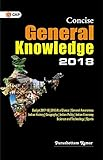# Time Domain Analysis – 1 – 5

21. Knowledge of the transfer function of a system is necessary for the calculation of :

(a) time constant
(b) output for a given input
(c) order of the system
(d) none of these

Explanation
No answer description available for this question. Let us discuss.

22. The steady – state error of a first – order system to a ramp input is equal to :

(a) the time constant of the system
(b) zero
(c) infinity
(d) none of these

Explanation
No answer description available for this question. Let us discuss.

23. For a second – order undamped system, the poles are :

(a) purely imaginary
(b) real and equal
(c) real and equal
(d) complex conjugate of each other

Explanation
No answer description available for this question. Let us discuss.

24. The transfer function is the ratio of :

(a) output to input
(b) the Laplace transform of output to the Laplace transform of input
(c) the Laplace transform of input to the Laplace transform of output
(d) the inverse Laplace transform of output to the inverse Laplace transform of input

Explanation
No answer description available for this question. Let us discuss.

25. Control systems are normally designed with damping factor :

(a) ξ = 0
(b) ξ = 1
(c) ξ > 1
(d) ξ < 1

Explanation
No answer description available for this question. Let us discuss.

General Knowledge Books##General Knowledge 2019 Price: Check on Amazon-17% General Knowledge Price: INR 179.00 Was: INR 216.00-43% Objective General Knowledge Chapterwise Collection of 6250+ Questions Price: INR 169.00 Was: INR 295.00Concise General Knowledge 2018 Price: INR 30.00General Knowledge 2018 (Old Edition) Price: Check on Amazon-16% Objective General Knowledge Price: INR 302.00 Was: INR 360.00 ‹›

Be a Part of the New & Next

Share the Knowledge

Copy Protected by Chetan's WP-Copyprotect.